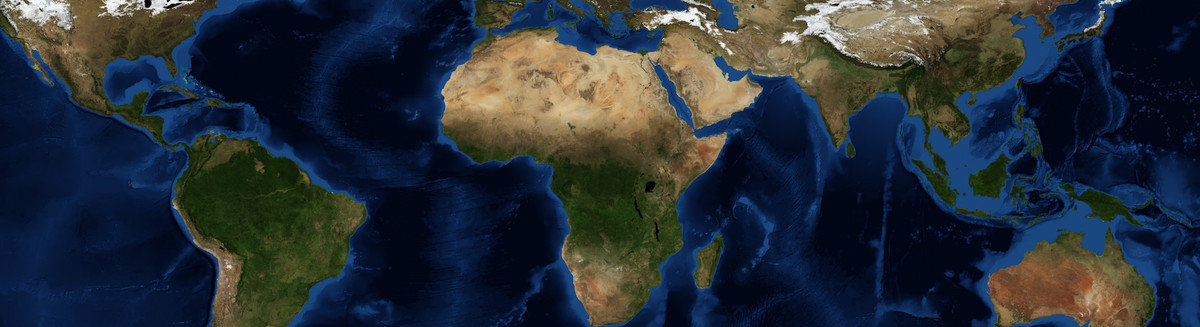This is a Preprint and has not been peer reviewed. This is version 1 of this Preprint.

##### Authors

Jean-Michel Brankart

##### Abstract

The purpose of this study is to further investigate the problem of explicitly simulating uncertainties in geophysical models. Rather than introducing stochastic processes in forward-in-time partial differential equations, this is done here by reformulating the dynamical equations governing the time evolution of the flow as a probability distribution. Flows can then be drawn directly from the probability distribution using an efficient sampler. This approach is illustrated in the paper with the example of a two-dimensional shallow-water flow on the surface of a rotating sphere. With the sampler, the simulated flows evolve smoothly in time according to the advection constraint, while time-dependent stochastic fluctuations are produced according to the probability distribution describing the effect of the unresolved motions. This can be done without closing the dynamical equations, with constraints applied only to what is known, and using a larger time step, since there is no more numerical stability condition to verify. From a physical point of view, this probabilistic reformulation introduces two main differences with respect to forward-in-time partial differential equations. First, dissipation can only behave symmetrically with respect to the reversal of time. It is shown however that the formulation can be made equivalent to the classic diffusion equation in the particular case of an initial condition problem. Second, in this framework, information about the past of the system does not need to be provided in the form of a complete initial condition at a given time. A constraint on the past of the system can here be directly applied using a history of incomplete and imperfect observations, thus resolving the inverse and direct problems together at a similar numerical cost.

##### DOI

https://doi.org/10.31223/osf.io/r83fa

##### Subjects

Earth Sciences, Fluid Dynamics, Physical Sciences and Mathematics, Physics

##### Dates

Published: 2020-06-19 13:39